Download Presentation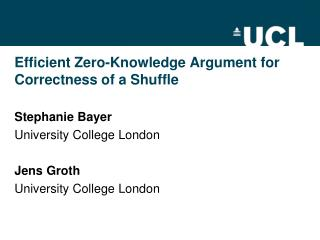Efficient Zero-Knowledge Argument for Correctness of a Shuffle

# Efficient Zero-Knowledge Argument for Correctness of a Shuffle - PowerPoint PPT Presentation

Efficient Zero-Knowledge Argument for Correctness of a Shuffle. Stephanie Bayer University College London Jens Groth University College London. Motivation – e-voting. Voting: - Voter casts secret vote - Authorities reveal votes in random permuted orderI am the owner, or an agent authorized to act on behalf of the owner, of the copyrighted work described.
Download Presentation## Efficient Zero-Knowledge Argument for Correctness of a Shuffle

An Image/Link below is provided (as is) to download presentation

Download Policy: Content on the Website is provided to you AS IS for your information and personal use and may not be sold / licensed / shared on other websites without getting consent from its author.While downloading, if for some reason you are not able to download a presentation, the publisher may have deleted the file from their server.

- - - - - - - - - - - - - - - - - - - - - - - - - - E N D - - - - - - - - - - - - - - - - - - - - - - - - - -
Presentation Transcript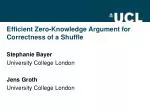### Efficient Zero-Knowledge Argument for Correctness of a Shuffle

Stephanie Bayer

University College London

Jens Groth

University College London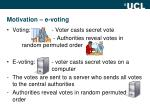Motivation – e-voting
• Voting: - Voter casts secret vote

- Authorities reveal votes in random permuted order

• E-voting: - voter casts secret votes on a computer
• The votes are sent to a server who sends all votes to the central authorities
• Authorities reveal votes in random permuted order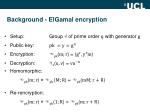Background - ElGamal encryption
• Setup: Group G of prime order with generator
• Public key:
• Encryption: E() = ()
• Decryption: D() =
• Homomorphic:
• E() ×E() = E()
• Re-rencryption:
• E() ×E() = E()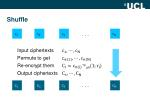Shuffle

. . .

Input ciphertexts

Permute to get

Re-encrypt them E()

Output ciphertexts

. . .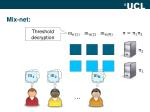Mix-net:

Threshold decryption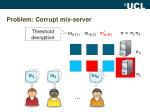Problem: Corrupt mix-server

Threshold decryption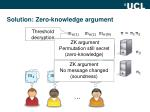Solution: Zero-knowledge argument

Threshold decryption

ZK argumentPermutation still secret(zero-knowledge)

ZK argumentNo message changed(soundness)

N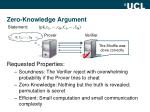Zero-Knowledge Argument

Statement: ()

Prover

Verifier

The Shuffle was done correctly

Requested Properties:

• Soundness: The Verifier reject with overwhelming probability if the Prover tries to cheat
• Zero-Knowledge: Nothing but the truth is revealed; permutation is secret
• Efficient: Small computation and small communication complexity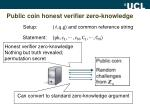Public coin honest verifier zero-knowledge

Setup: (G,,) and common reference string

Statement: ()

Honest verifier zero-knowledgeNothing but truth revealed; permutation secret

Prover Verifier

Can convert to standard zero-knowledge argument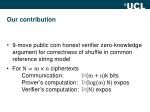Our contribution
• 9-move public coin honest verifier zero-knowledge argument for correctness of shuffle in common reference string model
• For ciphertexts
• Communication: O()k bitsProver’s computation: O() exposVerifier’s computation: O() exposCommitments
• Commit to a column vector Z as A=com()
• Length reducing
• Computational binding
• Perfectly hiding
• Homomorphic
• com(;)*com(;) = com(; )
• Pedersen Commitment: com(;) =Techniques - Sublinear cost
• Length reducing commitments
• Batch verification
• Structured Vandermonde challenges

Sublinear communication costShuffle argument
• Given public keys and
• Given ciphertexts and
• Prover knows permutation and randomizers and wants to convince the verifierE() E()Shuffle argument

The prover commits to a permutation by committing to

• Verifier sends challenge Z

The prover commits to

The prover gives an argument that both commitments are constructed using the same permutation

The proverdemonstrates that the input ciphertexts are permuted using the same permutation and knowledge of the randomizers used in the re-encryption.Shuffleargument
• Prover commits to as
• A=com()=com()
• and after receiving challenge Z to
• B= com() =com(s)

Both polynomials are equal, only the roots are permuted

InexpensiveSee full paper

• Prover gives product argument for A, B such that
• =

ExpensiveWill sketch idea

• Sketch idea focusing on soundness
• Ignore ZK (easy and cheap to add)
• Will also for simplicity assume randomnessNotation
• B contains commitments B, , Bwhere
• B= com=com(), , B= com ()
• Arrange ciphertexts in matrix
• =
• Define inner product = to simplify the statement asMulti-exponentiation argument

Communicaton:O() elements

Verifier computation: + O() expos

Prover sends

2ciphertexts

• Verifier sends challenge Z
• Prover opens
• to

elements in Zq

ciphertext expos

• Verifier computes and checks

ciphertext expos

ciphertext exposProver’s computation

Computingthis matrix costs m2n = mNciphertextexposReducing the prover’s computation
• Do not compute entire matrix
• Instead use techniques for multiplication of polynomials “in the exponent” of ciphertexts
• Fast Fourier Transform
• O(N log m) exponentiations O (1) rounds
• Interaction
• O (N) exponentiations O (log m) roundsImplementation
• Implementation in C++ using the NTL library and the GMP library
• Different levels of optimization
• Multi-exponentiation techniques
• Fast Fourier Transform
• Extra Interaction and Toom-CookComparison
• Runtime comparison of Verificatum (Wikström) to our shuffle argument
• MacBook Pro; CPU: 2.54 GHZ, RAM: 4GB
• , 60
• ciphertexts,